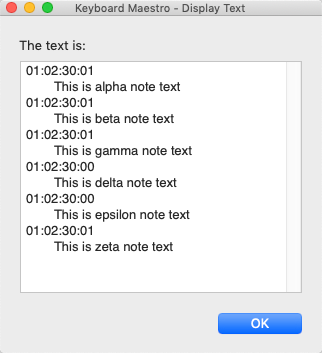# Best way to reformat this type of string…

Hi,

I need to design a macro that does 2 things but don’t know where to start.

I receive notes from a client that are written in a google doc. Each note begins with timecode and is followed by the actual note. For example:

01:02:30:01 - This is example note text.

The timecode format is hours:minutes:seconds:frames. Aka - HH:MM:SS:FF

Parsing
The macro needs to be able to parse the timecode from the note and create 2 corresponding variables, one var for the timecode, a second var for the note itself. So the example would result in a parse like this:

Timecode - 01:02:30:01
Note - This is example note text

Timecode Formatting
The other thing that needs to happen is to make sure the timecode is formatted correctly.

For my purposes, the timecode must be in the format in my example: HH:MM:SS:FF But oftentimes the timecode will be written like this:

02:30, I.e., MM:SS
2:30 ie, M:SS
O2:30;01 ie, MM:SS:FF (note use if senicolon before FF)

If not otherwise specified, the first two digits can always be assumed to be 01, and the last two can always be 00.

So the logic would be something like:

If the timecode format is xx:xx:xx:xx, use as is

If timecode includes semi colon. Replace with colon

If format is xx:xx, prepend with 01:, and append with :00

If format is x:xx, prepend with 00:0 and append with :00.

….

The input is a list of such lines ?

(or just one line ?)

On approach might be to use a Keyboard Maestro Execute JavaScript for Automation action:

This rough draft assume there there might be one or more such lines:

Timecode and note.kmmacros (4.4 KB)Expand disclosure triangle to view JS Source
``````(() => {
"use strict";

// @RobTrew 2022
// Normalized time-codes
// First sketch Ver 0.01

const main = () => {
const
kme = Application("Keyboard Maestro Engine"),
xs = lines(kme.getvariable("incomingLines"));

return xs.flatMap(x => {
const s = x.trim();

return s.includes("-") ? (() => {
const
[code, note] = s.split(/\s-\s/u)
.slice(0, 2),
digitStrings = code
.replace(/[Oo]/ug, "0")
.split(/[:;]/ug),
nDigits = digitStrings.length,
rawValues = digitStrings.map(
v => parseInt(v, 10)
);

const fullValues = (4 === nDigits) ? (
rawValues
) : .concat(
rawValues.concat(
replicate(3 - nDigits)(0)
)
),
delimited = fullValues.map(
).join(":");

return [
`\${delimited} - \${note}`
];
})() : (
Boolean(s) ? (
[`No hyphen seen in '\${s}'.`]
) : []
);
})
.join("\n");
};

// --------------------- GENERIC ---------------------

// lines :: String -> [String]
const lines = s =>
// A list of strings derived from a single string
// which is delimited by \n or by \r\n or \r.
Boolean(s.length) ? (
s.split(/\r\n|\n|\r/u)
) : [];

// replicate :: Int -> a -> [a]
const replicate = n =>
// A list of n copies of x.
x => Array.from({
length: n
}, () => x);

return main();
})();
``````

@Djh you wrote:

SO I would guess this is a mistake on your part:

`If format is x:xx, prepend with 01:0 and append with :00`